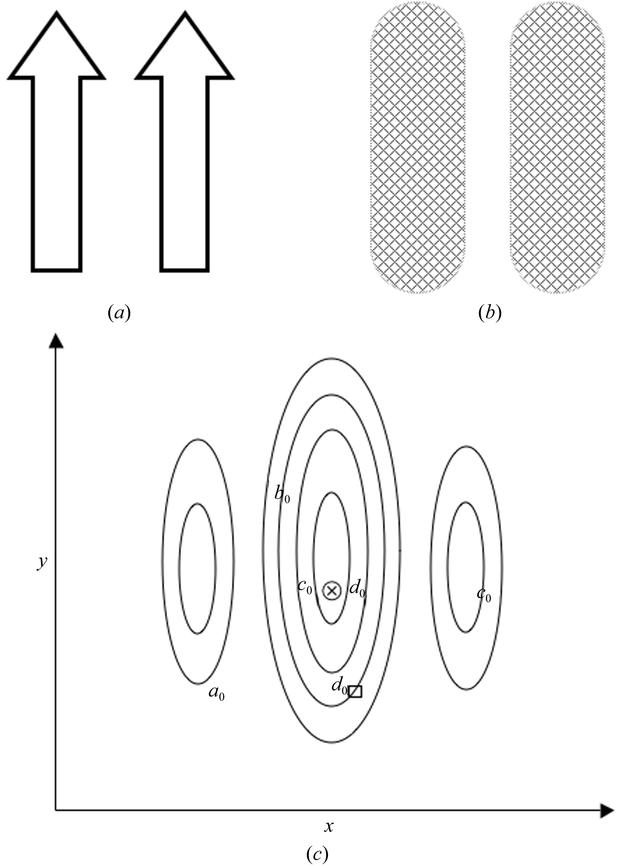disable zoom     view article Figure 1 A hypothetical example of a problem with several local maxima. In this problem, a two-stranded ribbon schematic (a) is being fit to a medium-resolution density map (b), an example of a two-dimensional search over the variables x and y. (c) shows the function landscape corresponding to the correlation between the model and the map over all values of the variables x and y. The function has three maxima: the middle correct one and two side maxima where only one strand of the two overlaps at about half the height of the center one. To illustrate how crossovers between two vectors in a GA can jump between local maxima or `hill-hop', imagine that we start a GA run with four vectors, a0, b0, c0 and d0, each with two genes x and y, assigned random values over the entire search space as shown in (c). If we make a daughter of c by crossing over between c and d such that we take the y value from c0 and the x value from d0, then the daughter that is produced will be very near the correct maxima and will replace the parent, `winning' over the other vectors.BIOLOGICALCRYSTALLOGRAPHY
ISSN: 1399-0047
Volume 60| Part 12| November 2004| Pages 2276-2279